# Assignments

Solve the assignments below. You can do this either by trying to determine a common divisor or by cross-multiplication. Which of the two is used depends on the nature of the assignment.

1. Solve: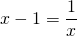Solution

2. Solve:Solution

3. Solve: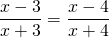Solution

4. Solve: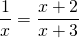Solution

5. Solve: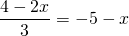Solution

6. Solve: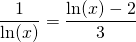Solution

7. For which value(s) of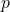does the following equation have two different solutions?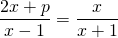Solution

8. Solve: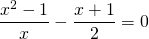Solution

9. For which value(s) ofdoes the following equation have no solutions: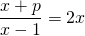Solution

10. Given the hyperbola: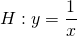and the line: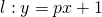For which value(s) ofis the line tangent to the hyperbola?

Solution

0